# Sacred Geometry Introduction

###Sacred Geometry Introductory Tutorial by Bruce Rawles

In nature, we find patterns, designs and structures from the most minuscule particles, to expressions of life discernible by human eyes, to the greater cosmos. These inevitably follow geometrical archetypes, which reveal to us the nature of each form and its vibrational resonances. They are also symbolic of the underlying metaphysical principle of the inseparable relationship of the part to the whole. It is this principle of oneness underlying all geometry that permeates the architecture of all form in its myriad diversity. This principle of interconnectedness, inseparability and union provides us with a continuous reminder of our relationship to the whole, a blueprint for the mind to the sacred foundation of all things created.

### The Sphere(charcoal sketch of a sphere by Nancy Bolton-Rawles)

Starting with what may be the simplest and most perfect of forms, the sphere is an ultimate expression of unity, completeness, and integrity. There is no point of view given greater or lesser importance, and all points on the surface are equally accessible and regarded by the center from which all originate. Atoms, cells, seeds, planets, and globular star systems all echo the spherical paradigm of total inclusion, acceptance, simultaneous potential and fruition, the macrocosm and microcosm.

### The CircleThe circle is a two-dimensional shadow of the sphere which is regarded throughout cultural history as an icon of the ineffable oneness; the indivisible fulfillment of the Universe. All other symbols and geometries reflect various aspects of the profound and consummate perfection of the circle, sphere and other higher dimensional forms of these we might imagine.

The ratio of the circumference of a circle to its diameter, Pi, is the original transcendental and irrational number. (Pi equals about 3.14159265358979323846264338327950288419716939937511…) It cannot be expressed in terms of the ratio of two whole numbers, or in the language of sacred symbolism, the essence of the circle exists in a dimension that transcends the linear rationality that it contains. Our holistic perspectives, feelings and intuitions encompass the finite elements of the ideas that are within them, yet have a greater wisdom than can be expressed by those ideas alone.

### The Point

At the center of a circle or a sphere is always an infinitesimal point. The point needs no dimension, yet embraces all dimension. Transcendence of the illusions of time and space result in the point of here and now, our most primal light of consciousness. The proverbial “light at the end of the tunnel” is being validated by the ever-increasing literature on so-called “near-death experiences”. If our essence is truly spiritual omnipresence, then perhaps the “point” of our being “here” is to recognize the oneness we share, validating all “individuals” as equally precious and sacred aspects of that one.

Life itself as we know it is inextricably interwoven with geometric forms, from the angles of atomic bonds in the molecules of the amino acids, to the helical spirals of DNA, to the spherical prototype of the cell, to the first few cells of an organism which assume vesical, tetrahedral, and star (double) tetrahedral forms prior to the diversification of tissues for different physiological functions. Our human bodies on this planet all developed with a common geometric progression from one to two to four to eight primal cells and beyond.

Almost everywhere we look, the mineral intelligence embodied within crystalline structures follows a geometry unfaltering in its exactitude. The lattice patterns of crystals all express the principles of mathematical perfection and repetition of a fundamental essence, each with a characteristic spectrum of resonances defined by the angles, lengths and relational orientations of its atomic components.

### The Square Root of Two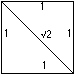The square root of 2 embodies a profound principle of the whole being more than the sum of its parts. (The square root of two equals about 1.414213562…) The orthogonal dimensions (axes at right angles) form the conjugal union of the horizontal and vertical which give birth to the greater offspring of the hypotenuse. The new generation possesses the capacity for synthesis, growth, integration and reconciliation of polarities by spanning both perspectives equally. The root of two originating from the square leads to a greater unity, a higher expression of its essential truth, faithful to its lineage.

The fact that the root is irrational expresses the concept that our higher dimensional faculties can’t always necessarily be expressed in lower order dimensional terms – e.g. “And the light shineth in darkness; and the darkness comprehended it not.” (from the Gospel of St. John, Chapter 1, verse 5). By the same token, we have the capacity to surpass the genetically programmed limitations of our ancestors, if we can shift into a new frame of reference (i.e. neutral with respect to prior axes, yet formed from that matrix-seed conjugation. Our dictionary refers to the word matrix both as a womb and an array (or grid lattice). Our language has some wonderful built-in metaphors if we look for them!

### The Golden Ratio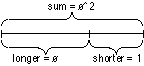The golden ratio (a.k.a. phi ratio a.k.a. sacred cut a.k.a. golden mean a.k.a. divine proportion) is another fundamental measure that seems to crop up almost everywhere, including crops. (The golden ratio is about 1.618033988749894848204586834365638117720309180…) The golden ratio is the unique ratio such that the ratio of the whole to the larger portion is the same as the ratio of the larger portion to the smaller portion. As such, it symbolically links each new generation to its ancestors, preserving the continuity of relationship as the means for retracing its lineage.The golden ratio (phi) has some unique properties and makes some interesting appearances:

• phi = phi^2 – 1; therefore 1 + phi = phi^2; phi + phi^2 = phi^3; phi^2 + phi^3= phi^4; ad infinitum.
• phi = (1 + square root(5)) / 2 from quadratic formula, 1 + phi = phi^2.
• phi = 1 + 1/(1 + 1/(1 + 1/(1 + 1/(1 + 1/(1 + 1/…)))))
• phi = 1 + square root(1 + square root(1 + square root(1 + square root(1 + square root(1 + …)))))
• phi = (sec 72)/2 =(csc 18)/2 = 1/(2 cos 72) = 1/(2 sin 18) = 2 sin 54 = 2 cos 36 = 2/(csc 54) = 2/ (sec 36) for all you trigonometry enthusiasts.
• phi = the ratio of segments in a 5-pointed star (pentagram) considered sacred to Plato and Pythagoras in their mystery schools. Note that each larger (or smaller) section is related by the phi ratio, so that a power series of the golden ratio raised to successively higher (or lower) powers is automatically generated: phi, phi^2, phi^3, phi^4, phi^5, etc.• phi = apothem to bisected base ratio in the Great Pyramid of Giza
• phi = ratio of adjacent terms of the famous Fibonacci Series evaluated at infinity; the Fibonacci Series is a rather ubiquitous set of numbers that begins with one and one and each term thereafter is the sum of the prior two terms, thus: 1,1,2,3,5,8,13,21,34,55,89,144… (interesting that the 12th term is 12 “raised to a higher power”, which appears prominently in a vast collection of metaphysical literature)

The mathematician credited with the discovery of this series is Leonardo Pisano Fibonacci and there is a publication devoted to disseminating information about its unique mathematical properties, The Fibonacci Quarterly

Fibonacci ratios appear in the ratio of the number of spiral arms in daisies, in the chronology of rabbit populations, in the sequence of leaf patterns as they twist around a branch, and a myriad of places in nature where self-generating patterns are in effect. The sequence is the rational progression towards the irrational number embodied in the quintessential golden ratio.

This most aesthetically pleasing proportion, phi, has been utilized by numerous artists since (and probably before!) the construction of the Great Pyramid. As scholars and artists of eras gone by discovered (such as Leonardo da Vinci, Plato, and Pythagoras), the intentional use of these natural proportions in art of various forms expands our sense of beauty, balance and harmony to optimal effect. Leonardo da Vinci used the Golden Ratio in his painting of The Last Supper in both the overall composition (three vertical Golden Rectangles, and a decagon (which contains the golden ratio) for alignment of the central figure of Jesus.

The outline of the Parthenon at the Acropolis near Athens, Greece is enclosed by a Golden Rectangle by design.

### The Square Root of 3 and the Vesica Piscis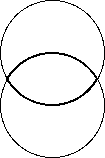The Vesica Piscis is formed by the intersection of two circles or spheres whose centers exactly touch. This symbolic intersection represents the “common ground”, “shared vision” or “mutual understanding” between equal individuals. The shape of the human eye itself is a Vesica Piscis. The spiritual significance of “seeing eye to eye” to the “mirror of the soul” was highly regarded by numerous Renaissance artists who used this form extensively in art and architecture. The ratio of the axes of the form is the square root of 3, which alludes to the deepest nature of the triune which cannot be adequately expressed by rational language alone.

### SpiralsThis spiral generated by a recursive nest of Golden Triangles (triangles with relative side lengths of 1, phi and phi) is the classic shape of the Chambered Nautilus shell. The creature building this shell uses the same proportions for each expanded chamber that is added; growth follows a law which is everywhere the same. The outer triangle is the same as one of the five “arms” of the pentagonal graphic above.

### Toroids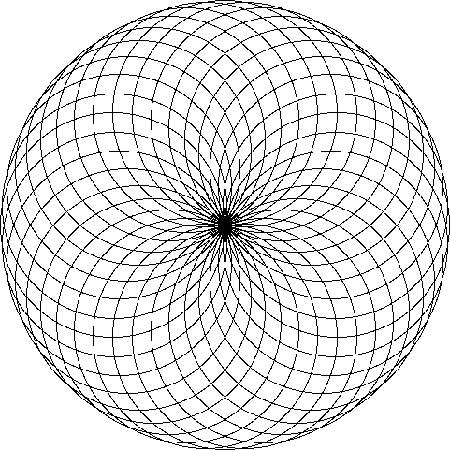Rotating a circle about a line tangent to it creates a torus, which is similar to a donut shape where the center exactly touches all the “rotated circles.” The surface of the torus can be covered with 7 distinct areas, all of which touch each other; an example of the classic “map problem” where one tries to find a map where the least number of unique colors are needed. In this 3-dimensional case, 7 colors are needed, meaning that the torus has a high degree of “communication” across its surface. The image shown is a “birds-eye” view.

### Dimensionality

The progression from point (0-dimensional) to line (1-dimensional) to plane (2-dimensional) to space (3-dimensional) and beyond leads us to the question – if mapping from higher order dimensions to lower ones loses vital information (as we can readily observe with optical illusions resulting from third to second dimensional mapping), does our “fixation” with a 3-dimensional space introduce crucial distortions in our view of reality that a higher-dimensional perspective would not lead us to?

### Fractals and Recursive GeometriesThere is a wealth of good literature on this subject; it’s always fascinating how nature propagates the same essence regardless of the magnitude of its expression…our spirit is spaceless yet can manifest aspects of its individuality at any scale.

### Perfect Right TrianglesThe 3/4/5, 5/12/13 and 7/24/25 triangles are examples of right triangles whose sides are whole numbers. The graphic above contains several of each of these triangles. The 3/4/5 triangle is contained within the so-called “King’s Chamber” of the Great Pyramid, along with the 2/3/root5 and 5/root5/2root5 triangles, utilizing the various diagonals and sides.

### The Platonic Solids

Here are LOTS of math details and images of the Platonic Solids and Archimedean Solids.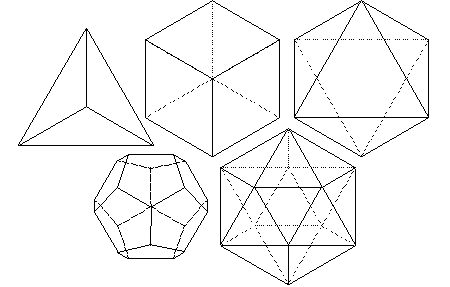The 5 Platonic solids (Tetrahedron, Cube or (Hexahedron), Octahedron, Dodecahedron and Icosahedron) are ideal, primal models of crystal patterns that occur throughout the world of minerals in countless variations. These are the only five regular polyhedra, that is, the only five solids made from the same equilateral, equiangular polygons. To the Greeks, these solids symbolized fire, earth, air, spirit (or ether) and water respectively. The cube and octahedron are duals, meaning that one can be created by connecting the midpoints of the faces of the other. The icosahedron and dodecahedron are also duals of each other, and three mutually perpendicular, mutually bisecting golden rectangles can be drawn connecting their vertices and midpoints, respectively. The tetrahedron is a dual to itself.

From April, 2000 through (at least) December, 2003, we conducted a poll on which Platonic Solid folks liked best; here’s the results from that period. If there’s interest, I’ll start another poll to see if the preferences has shifted!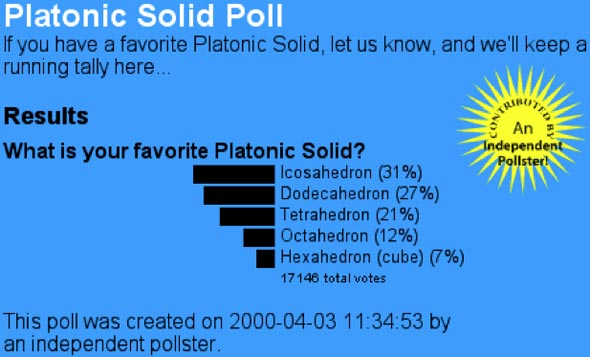### The Archimedean SolidsThere are 13 Archimedean solids, each of which are composed of two or more different regular polygons. Interestingly, 5 (Platonic) and 13 (Archimedean) are both Fibonacci numbers, and 5, 12 and 13 form a perfect right triangle.

### Stellations of The Platonic Solids and The Archimedean SolidsThis is a stellation of a dodecahedron where each pentagonal face is capped with a pentagonal pyramid composed of 5 golden triangles, a sort of 3-dimensional 5-pointed star.

Here are more images of polyhedra (Platonic and Archimedean Solids.)

### Metatron’s Cube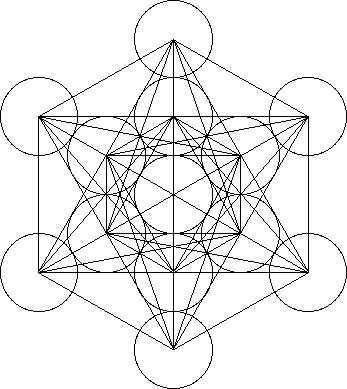Metatron’s Cube contains 2-dimensional images of the Platonic Solids (as shown above) and many other primal forms.

### The Flower of LifeIndelibly etched on the walls of temple of the Osirion at Abydos, Egypt, the Flower of Life contains a vast Akashic system of information, including templates for the five Platonic Solids. The background graphic for this page is a repetitive hexagonal grid based on the Flower of Life. Below is an example of Flower of Life jewelry.